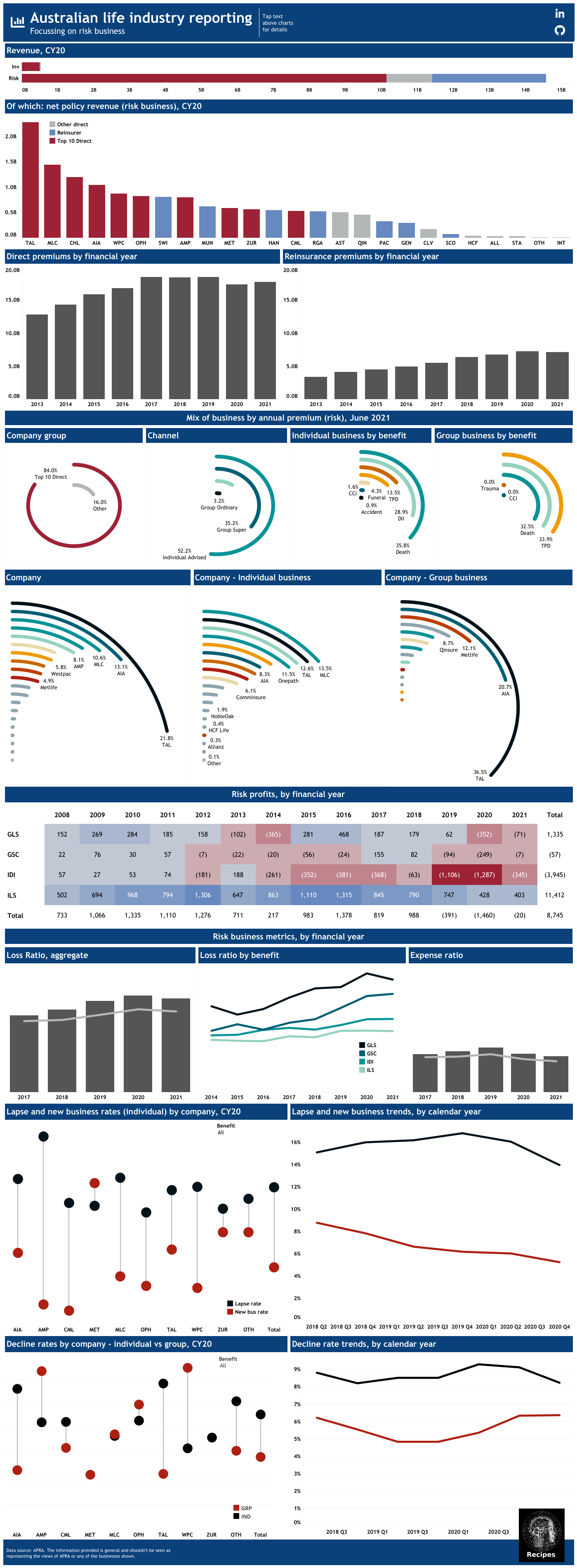# R: Life Industry Stats in Tableau and R#

By Pat Reen - originally published on his GitHub site here, which has the original code.

# Overview#

APRA release Australian life industry data on performance quarterly at a product level as well as aggregated results by company. Bi-annually APRA release other statistics on lapse rates, new business rates, decline rates and market share. This section presents a visualisation of these datasources in Tableau. Also included is an R script which could be used to extract results from the APRA data.

Tableau public is a free to use visualisation tool that can ingest data in a number of different formats and is useful at creating flexible visualisation.

See link above to GitHub repository which has data and tableau workbook for this recipe.

A radial bar chart is a bar chart plotted in polar co-ordinates rather than a Cartesian plane. This site sets out a very simple approach, which are used here.

# The result#

A preview of the final visualisation is below (click through to Tableau):# Using r to view industry stats#

As with most data sources, an alternative visualisation tool is R. The below extracts the individual disability income profits and revenue and calculates the margin.

not_run_sample_only <- function(){
# this script reads in the APRA data and produces a few graphs for presentation

packages <- c("tidyverse", "ggplot2", "readxl", "lubridate", "scales", "xtable")
install.packages(setdiff(packages, rownames(installed.packages())))
for (package in packages) {
library(package, character.only = TRUE)
}

percent0 <- function(value) {
return(percent(value, accuracy = 0.1))
}

# update names
#  "Reporting date"      "Industry sector"     "Subject"
#  "Category"            "Data item"           "Reporting Structure"
#  "Class of business"   "Product Group"       "Calculation basis"
#  "Value"               "Notes"

qrt_col_name <- c(
"rep_date", "sector", "subject", "category", "data_item",
"rep_struc", "class", "product", "calc_basis", "value",
"notes"
)
qrt_col_type <- c("date", rep("text", 8), "numeric", "text")

# update to relevant path
path = "Quarterly life insurance performance statistics database - June 2008 to June 2021.xlsx",
sheet = "Data",
col_names = qrt_col_name,
skip = 1,
col_types = qrt_col_type,
trim_ws = TRUE, na = "N/A"
)

qrt_data$fin_year <- paste0("FY", format(year(qrt_data$rep_date) +
as.integer(month(qrt_data$rep_date) > 6))) # also add calendar year as an alternative aggregation qrt_data$cal_year <- format(year(qrt_data$rep_date)) # DI Profit by Year ----------------------------------------------------- DI_risk_type <- "Individual Disability Income Insurance" data_items <- c( "Profit / loss before tax ($m)" = "Profit / loss before tax",
"Premiums after reinsurance ($m)" = "Net policy revenue", "Premiums before reinsurance ($m)" = "Gross policy revenue"
)

DI_profit <- qrt_data %>%
filter(data_item %in% data_items) %>%
filter(is.na(class)) %>%
filter(product == DI_risk_type) %>%
group_by(Fin year = fin_year, data_item) %>%
summarise(risk_value = sum(value)) %>%
mutate(Margin (%) = percent0(Profit / loss before tax /
Net policy revenue)) %>%
rename(data_items)

DI_profit_print <- xtable(
x = DI_profit,
caption = "Individual Disability Income Industry Profit",
align = "llrrrr",
digits = 0
)

print(DI_profit_print,
type = "html",
file = "DI_profit",
include.rownames = FALSE,
)
}